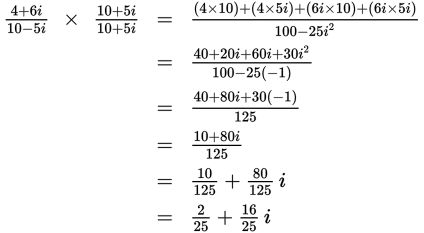# SAT Math Multiple Choice Question 949: Answer and Explanation

### Test Information

Question: 949

1. Which of the following is equivalent to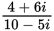? (Note: i2 = -1)

• A.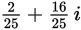• B.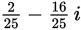• C.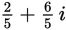• D.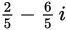Explanation:

A

Difficulty: Medium

Category: Additional Topics in Math / Imaginary Numbers

Strategic Advice: Because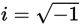, you can't leave an i in the denominator of a complex number (it's not considered proper notation to leave a radical in a denominator). This means you'll need to rationalize the denominator by multiplying the numerator and denominator by the conjugate of the denominator. The conjugate of (a + bi) is (a - bi). In other words, keep the real part the same and change the sign of the imaginary part.

Getting to the Answer: The conjugate of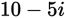is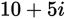; multiply the numerator and denominator of the given expression by this to remove i from the denominator. Use FOIL to expand the binomial pairs, and then combine like terms to simplify. Separate the real and imaginary parts and reduce as needed. The steps for this sequence are shown here: